Greetings,

While I have asked a couple Group By questions on here, I have a final one that is proving to be challenging.

@ppr has been a tremendous resource, and I am hoping he can chime in one last time to help me with this.

What I am trying to accomplish:

Group by:

``````(From d in dtData.AsEnumerable
Group d by k=d("Extended Price").toString.Trim into grp-Group
``````

So i have that part figured out.

Now, I need:

• For each row, in each group
• Apply the following formula under row.field(“quantity”) - CInt(Math.Ceiling(“quantity”)/0.9)
• Then, after that formula has been applied (basically dividing the value under the row field “quantity” by .9 and then rounding the answer up to the nearest whole number (example: 104.78 rounded up to 105.00), apply the sum method.

So, for each row in each group, apply that division and then round up method, and then sum all those up, and save them to a datatable.

I know that the rest of the code is going to be somewhat like this:

``````For each row - ??

Let cs = CInt(Math.Ceiling("quantity")/0.9) - ??
Let cn = grp.Sum(Function (cs) CInt( "0" & cs("Quantity").toString)) - ??
Let ra = New Object(){k, cs, cn}
``````

I know I am close. This is the result ChatGPT gave me:

``````dtData.AsEnumerable()
GroupBy(Function(d) d("Extended Price").ToString().Trim())
Select(Function(grp)

New With {
k = grp.Key,
cs = grp.Sum(Function(rc)
CInt(Math.Ceiling(rc("quantity")/0.9))),

.CopytoDataTable
``````

Thank you very much in advance (especially @ppr! )

@Sc100

`(From d In DtBuild.AsEnumerable() Group d By k=d("Extrnded Price").toString.Trim Into grp = Group Let sum = grp.Sum(function(x) Math.Ceiling(Cdbl(x("quantity").ToString)/0.9)) Let ra = New Object(){k,sum} Select r = DtClone.Rows.Add(ra)).CopyToDataTable()`

Cheers

Hi Anil,

Thank you so much for your response. That code does successfully compile!

I am getting an error, though. It is saying the columns are not found. I think I am missing one last final detail.

The following screenshot is a visual of what the spreadsheet would look like after filtering - so I am thinking - instead of grouping by “column”, should it be by “row field”?

So, using your code: (I changed the group by and sum column names, by the way)

``````(From d In DtBuild.AsEnumerable() Group d By k=d("Line").toString.Trim Into grp = Group
Let sum = grp.Sum(function(x) Math.Ceiling(Cdbl(x("Extended Net Price (USD)").ToString)/0.9))
Let ra = New Object(){k,sum}

``````

To reiterate and clarify - I am getting an error saying column “Line” is not found in datatable, and column “Extended Net Price (USD)” is not found in datatable.

Could you kindly tell me the changes I would need to make within the code to indicate row field vs column? (if that is indeed what needs to be changed)

Really appreciate the help!

@Sc100

I did not fully understand what you are trying to do now…have qfew questions first being …is that column header repeating many times?

And what you want to group as I see line is not repeating there is no need to group…

And now coming to the error it says the column names provided are missing in the input datatable…

Cheers

Anil,

“Is that column header repeating?” - Yes

“What you want to group” - I chose “Line” as somewhat arbitrary, but basically I need everything within the dark, repeating header that contains (“Line”, etc, etc") separated as a group, and within each group, I just need to apply the division and round up formula to each individual cell in the “Extended Net Price (USD)” column header and then get the sum total.

Referencing code from another automation that I am thinking would help get closer…I would just need to add the Group by, and then for each group, apply something similar to the below code: (and of course, replace “Ext MRC” with “Extended Net Price (USD)”

``````Convert.ToString(buildData.AsEnumerable().Sum(Function(row)Convert.ToDouble(I
f(String.IsNullOrEmpty(Convert.ToString(row("Ext MRC"))),"0",Convert.ToString(row("Ext MRC"))))))

``````

With the above code, “buildData” was a datatable built via the build datatable method.

As I am thinking - would it be better if I first Group By "Extended Net Price (USD), and have each group saved - as an array - to 1 column datatable? So then, each row in the datatable corresponds to a group, which is just an array of the prices, and from there, for each array item, apply the division and rounding up, and then finally sum.

Thank you for you patience in helping with this!

I hope this makes more sense - please let me know if you need any other clarification.

refering to the shown excel

a LINQ groupBy on using a particular column will not group the data as we do see it within the excel.

We would recommend:

• calculate the start/end index of each block
OR
• use a helper column and add on each row group counter mark

here we would need more details as the expected output / resulting datatable is unclear

We would also recommend to check the need for a LINQ as implementing it with the help of a for each row could also be a valid alternative.

Thank you, Peter

If LINQ cannot group by the blocks in the excel file, that is fine. I am happy with any solution.

The expected output would be, for each block:

To essentially get the sum of all the values under the “Extended Net Price (USD)” column (circled in red), and save them to a datatable looking something like:

Column1 (Block name) | Column2 (Sum)

Block 1| 100
Block2 | 150
Block 3| 100

etc, etc

To calculate a start/end index for each block, assuming that the blocks can vary in size - What would be the best way to approach this? LINQ or with activities?

Would really appreciate a quick run through of how you would approach this using:

• start/end index for each block
• For each row in each block

Open to all solutions.

Thank you again!

Variables:

Sample data (here we react on Line as SplitText)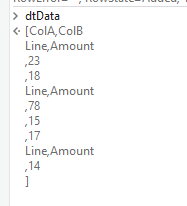Flow for getting the group Member Rows

arrTriggerIndex =

``````(From i In Enumerable.Range(0, dtData.Rows.Count)
Where dtData.Rows(i)(0).ToString.Trim.Equals(strSplitTrigger)
Select x = i).toArray
``````
``````arrTriggerIndex.Select(Function (x) x +1).toArray
arrSkippers.Zip(arrSkippers.Skip(1).Append(dtData.Rows.Count + 1), Function (x,y) new Int32(){x,y - x - 1}).ToList
ListSkipTakePairs = arrSkippers.Zip(arrSkippers.Skip(1).Append(dtData.Rows.Count + 1), Function (x,y) new Int32(){x,y - x - 1}).ToList
``````

Inspections: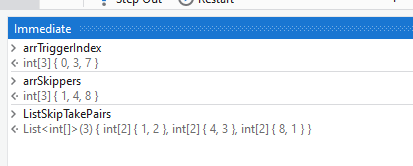Loop Inspections: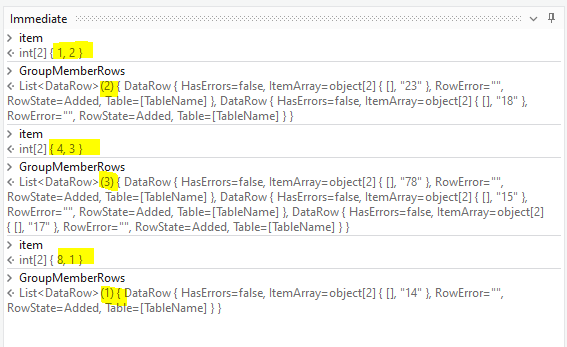As An alternate you can also modify the following approach

1 Like

Peter,

Absolutely outstanding reply from you, my friend.

I will dig into this and experiment.

Is there any way/anywhere I can send you a tip/cup of coffee?Let me know!

1 Like

@Sc100

You can as well use find /replace activity to first find the cells with headers…so that you know the start and end index of each set…then we can read the data from only those rows and sum them…

Cheers

@ppr

I spent a couple hours to construct, analyze, and test what you gave me. I understand how it works, and I thank you again for taking the time to share the steps. However, I have a couple questions remaining.

1. All the code for the variables are compiling successfully, but it looks like you may have left out the code for the “GroupMemberRows” variable.

Could you kindly provide that?

2. When I run debug mode to test the other variables (I have the for each item in ListSkipPairs disabled for now), I am not getting any results: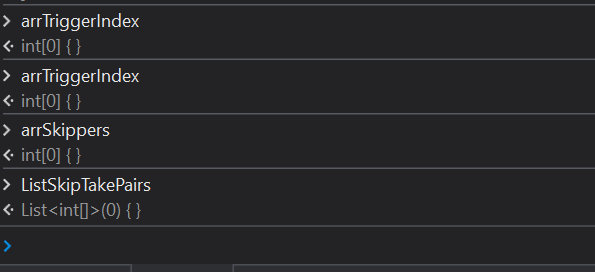This is the immediate view of my datatable, just for reference. It is identical to the one you showed: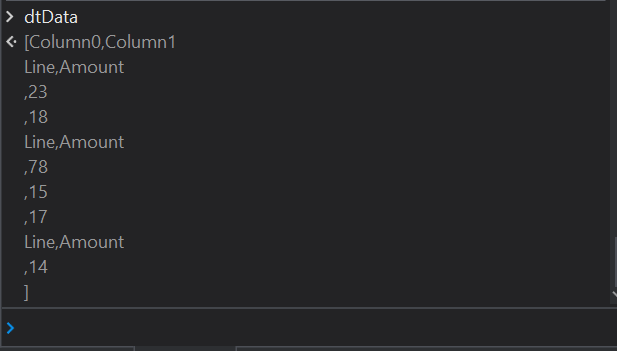I have attached the .xaml file as well as the excel file I used for read range/datatable.
Index_Skip_Lab.xaml (11.8 KB)
dtforLab.xlsx (8.6 KB)

Thank you again, sir!

``````dtData.AsEnumerable.skip(item(0)).Take(item(1)).tolist
``````
1 Like

Thank you, that code works for GroupMemberRows -

I am still getting the following for the variables: